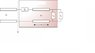# Impedance matching and Smith diagram.

• peripatein
It looks correct. You can find a more detailed explanation of how to solve this problem in the book Microwave Engineering by David Pozar.I have asked my instructor for clarification on the "cutoff stubs" and it appears the question asks for a way to realize Z2 and Y1 using two stubs which have a cutoff at their extremities, i.e. zero admittance.

#### peripatein

May I pose a question concerning impedance matching and Smith diagrams?

If you don't have a hurry i can take look.

I'd certainly be grateful :-).
In order to match (impedance wise) a transmission line without any losses to a load with impedance ZL the matching network is used, as shown in the diagram attached. Both Z2 and Y1 in the diagram are pure imaginary and connected in parallel and in series (as shown) to a transmission line of length d. It is stated that the matching network cannot match a load with admittance whose real part is greater than 1.6. I am then asked to use a Smith chart to determine the possible values of d. I did that, hopefully successfully, and obtained d=0.133λ, 0.383λ. The question then states the possible values of d to be d1=0.4λ and d2=0.1λ. The normalized Y1 and Z2 of the network are also given, as Y1=1.2j and Z2=1.2j. At this point I am asked to find all the possible values of the normalized admittance YL for which a perfect matching is obtained at point A in the diagram.
I am not really sure how to determine that using the Smith chart. I have tried writing the following equation, but I am not sure it'd get me somewhere:
YinA=1=Y2+YinT+Y1+YL, all normalized of course and where YinT refers to the transmission line.
I'd appreciate some guidance.

#### Attachments

•Untitled.jpg
11.7 KB · Views: 490
I try to solve for d=0.1λ. If it is correct you can follow same procedure.

Firts, if there is a match, impedance of point A should be 1.
Now, we can find impedance of point B(please check attachments).
ZB=ZA-Z2=1-1.2j

Now, show this impedance on the chart. Wave length of this point is 0.168λ(towards the load). If we turn 0.1λ towards the load we can find impedance of point C.

ZC=3+1.8j . YC=(1)/(ZC)

YC=0.311-0.083j
YC=YL+Y1
YL=0.311-1.28j

I hope this is helpful :)

#### Attachments

Would it please be possible for you to take better shots of the two sheets (calculations+chart)? It is very difficult to read them.

It looks great, thank you so, so much!
The last section of the question says that Y1 and Z2 are now implemented via cutoff stubs of length l1 and l2 respectively. I am asked to find the shortest lengths. What are cutoff stubs? Are they stubs with cutoff at their extremity (instead of, let's say, short)?

I don't know what is a cutoff stub. For double stub matching you can check the book Microwave Engineering by David Pozar.

I have asked my instructor for clarification on the "cutoff stubs" and it appears the question asks for a way to realize Z2 and Y1 using two stubs which have a cutoff at their extremities, i.e. zero admittance. I hence tried to move towards the generator along the circle r=0 from the leftmost extremity in the chart (where Y=0) to Y1 (=1.2j). The distance was found to be 0.14λ. I then moved along the same path until I reached Y2=-0.83j, a distance equal to 0.39λ. I therefore concluded that these should be the lengths of the stubs used in order to realize Z2 and Y1. Does that seem correct to you (my approach and numerical values obtained)?